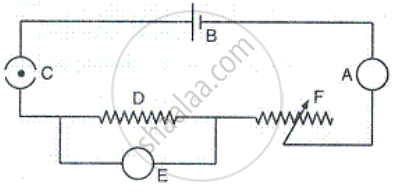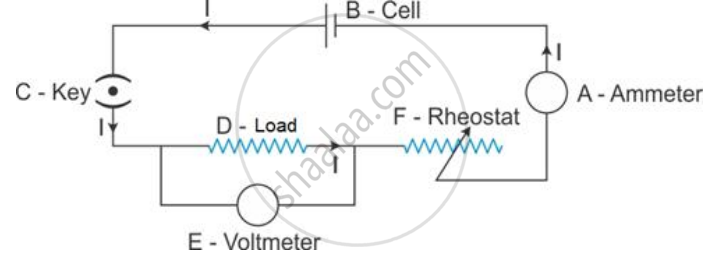# In the Electric Circuit Shown in Figure, Label the Parts A, B, C, D, E, and F. State the Function of Each Part. Show in the Diagram the Direction of Flow of Current. - Physics

In the electric circuit shown in Figure, label the parts A, B, C, D, E, and F. State the function of each part. Show in the diagram the direction of flow of current.#### Solution

A: Ammeter - It measures the current flowing through the circuit.

B: Cell - It acts as a source of direct current for the circuit.

C: Key - It is used to put the current on and off in the circuit.

D: Load - It is an appliance connected in a circuit. It may just be a resistance (e.g., bulb) or a combination of different electrical components.

E: Voltmeter - It is used to measure the potential difference between two points of a circuit.

F: Rheostat - It is used to control the current in the circuit.Concept: Simple Electric Circuit Using an Electric Cell and a Bulb to Introduce the Idea of Current
Is there an error in this question or solution?

#### APPEARS IN

Selina Concise Physics Class 9 ICSE
Chapter 9 Current Electricity
Exercise 9 (A) | Q 14 | Page 193